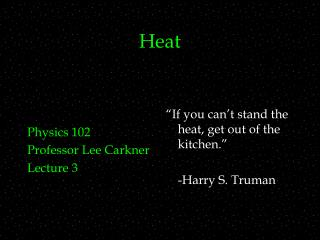DownloadDownload PresentationHeat

# Heat

Download Presentation## Heat

- - - - - - - - - - - - - - - - - - - - - - - - - - - E N D - - - - - - - - - - - - - - - - - - - - - - - - - - -
##### Presentation Transcript

1. Physics 102 Professor Lee Carkner Lecture 3 “If you can’t stand the heat, get out of the kitchen.” -Harry S. Truman Heat

2. PAL #2 Galileo Thermometer • How does it work? • Limitations

3. Heat • What is heat? • Same temperature, no heat • Heat used to be thought of a fluid (caloric) that could flow to change temperature • Heat is represented by the letter Q

4. Measuring Heat • Common unit of heat is the calorie: • Amount of heat necessary to increase the temperature of 1 gram of water by 1 C • In nutrition the Calorie is used • Case sensitive! • For rates of heat transfer (Q/t), unit is the Watt (W)

5. Heat and Temperature • If you heat a metal spoon and a wooden spoon for the same time, which will have a higher T? • The specific heat

6. Specific Heat • The specific heat is defined as: • c has units of J/kg C • Need to know the mass of the stuff (m) and the change in temperature (DT) Q =mcDT

7. Today’s PAL • A certain amount of heat Q will warm 1 g of material A by 3 degrees C and 1 g of material B by 4 degrees C. Which material has the greater specific heat? Explain.

8. Calorimetry • Insulated container that prevents heat transfer from outside • Since calorimeter is insulated, negative heat lost cancels out positive heat gained • Q1 + Q2 + Q3 … = 0 • Heat gained always positive, heat lost always negative • Make sure units for T and m match units for c

9. Example: Quenching a Dagger • Suppose a silver dagger of mass ms at Ts is immersed in a mass mw of water at Tw. What is the final temperature of the water? Qsilver + Qwater = 0 csmsDT + cwmwDT = 0 csms(Tf - Ts) + cwmw(Tf- Tw) = 0 csmsTf -csms Ts + cwmwTf - cwmw Tw = 0 csmsTf + cwmwTf = csms Ts + cwmw Tw Tf = (csms Ts + cwmwTw)/(csms+ cwmw)

10. How Does Heat Move? • Heat (like information) is transferred in different ways • Conduction • Radiation • Convection

11. Conduction • Why? • They interact and collide with other atoms and electrons and pass the energy on

12. Conduction Rate Factors • Free electrons • Density • Cross sectional area • Large window loses more heat than small • Temperature difference • Thickness • Heat takes less time to move through thinner material

13. Radiation • How does the energy from the Sun get to Earth? • How can energy be transported with no physical contact? • Photons are emitted by the Sun and absorbed by you • All objects emit photons

14. Radiation Rate Factors • Surface area • Emissivity • Radiation is strongly dependant on T

15. The Surface of the Sun

16. Convection • Hot air is less dense than the cooler air above it • After cooling the air may fall back down • Examples: baseboard heating, boiling water, Earth’s atmosphere

17. Convection Rate Factors • Fluidity • Energy exchange with environment • ? • How rapidly will the material lose heat? • Small temperature difference, not enough density difference to move

18. Today’s PAL • A hot piece of metal is at the bottom of a canister that can be completely filled with: • solid iron • liquid water • air • a vacuum • Consider the heat flow from the bottom to the top. • In which situation(s) would there be no conduction? • In which situation(s) would there be no convection? • In which situation(s) would there be no radiation?

19. Conduction Diagram A T2 T1 Q L

20. Conductive Heat Transfer • The rate of heat transfer via conduction is: • where: • T1 is the temperature of the hot side and T2 is the temperature of the cold side • A is the cross sectional area • L is the thickness • k is the thermal conductivity • High k = large heat transfer • Low k = small heat transfer

21. Radiative Heat Transfer • The amount of heat radiated out from an object is called the power (P): • where • s = the Stefan-Boltzmann constant • 5.6696 X 10-8 W/m2 K4 • A is the surface area • e is the emissivity (number between 0 and 1) • 0 = • perfect reflector • 1 = • perfect absorber or black body

22. Radiation Exchange • All objects emit and absorb radiation Pnet = sAe(T4-T42) • Where T2 is the temperature of the surroundings • Note that T must be in Kelvin

23. Next Time • Read: 13.6-13.11 • Homework: CH 14, P: 13, 47, CH 13, P: 29, 48 • Help sessions start next week • Tuesday and Thursday 6-8pm Science 304